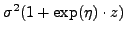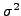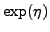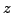Next: Mapproj package (mapproject) problems Up: Spatial and spatio-temporal data Previous: Using gamm()

#### User-defined variance functions in gamm() and lme()

One can set up a heteroscedastic residual variance structure by defining one's own variance function. Call gamm() or lme() as follows:
uMod=gamm(y~x,weights=varSum(form=~z))

Here, the variance is a function of a variable, z. To create the desired variance function, referred to as varSum above, one needs this code , which you can modify to create your own function by modifying line 75. In this particular example, the form of the variance is(see line 75). Note that the code uses the inverse of the standard deviations as the weights, and thatis the usual residual variance output from gamm() or lme(). Also note that I useto ensure that the second parameter in the variance function is non-negative.

This example variance function is useful if one is fitting a secondstage model in which the heteroscedasticvariance from the first stage () is added to additional homoscedastic variance, withserving to weight the two variance components, whilescales the overall variance.

Last modified 12/28/07.Next: Mapproj package (mapproject) problems Up: Spatial and spatio-temporal data Previous: Using gamm()
Chris Paciorek 2012-01-21